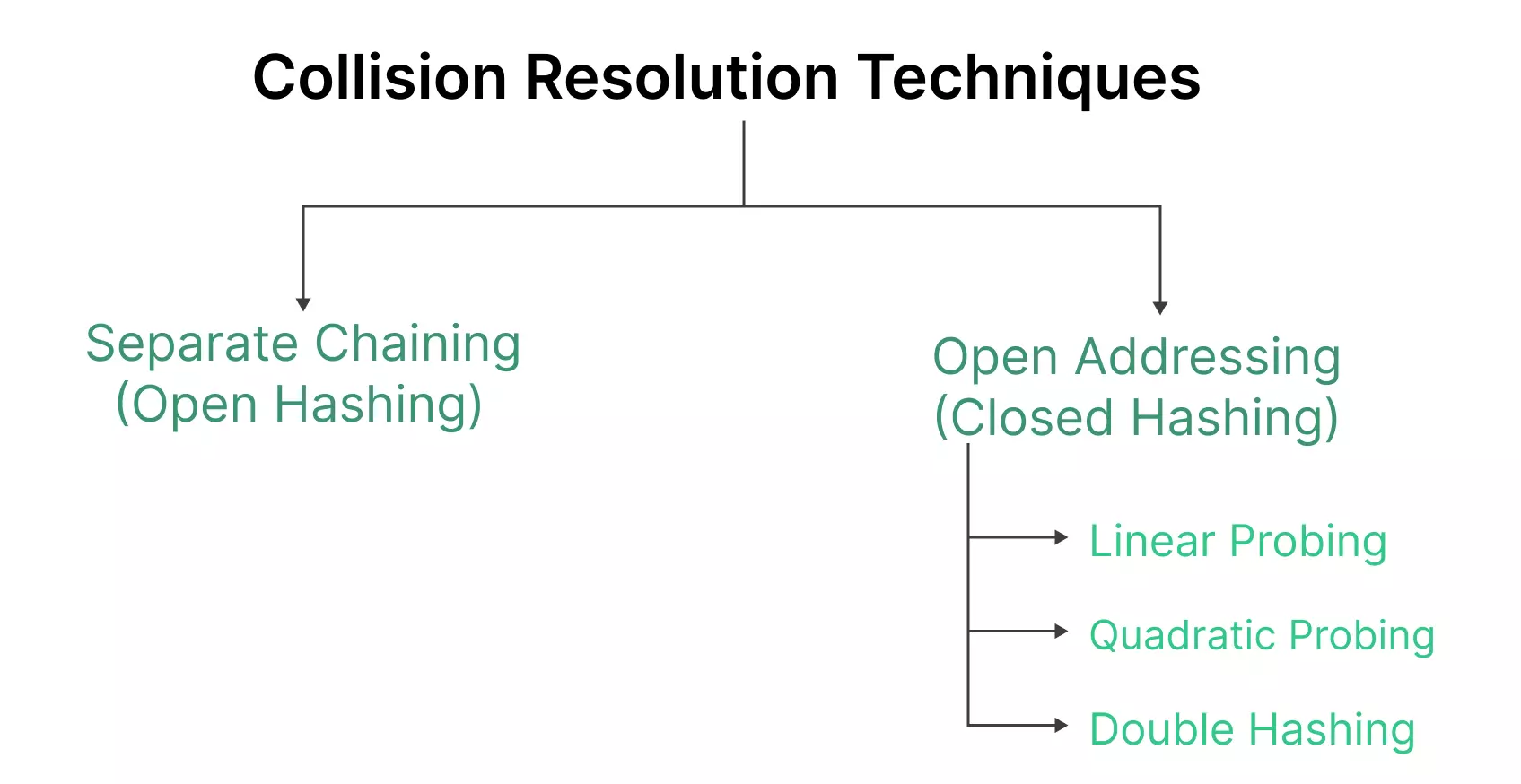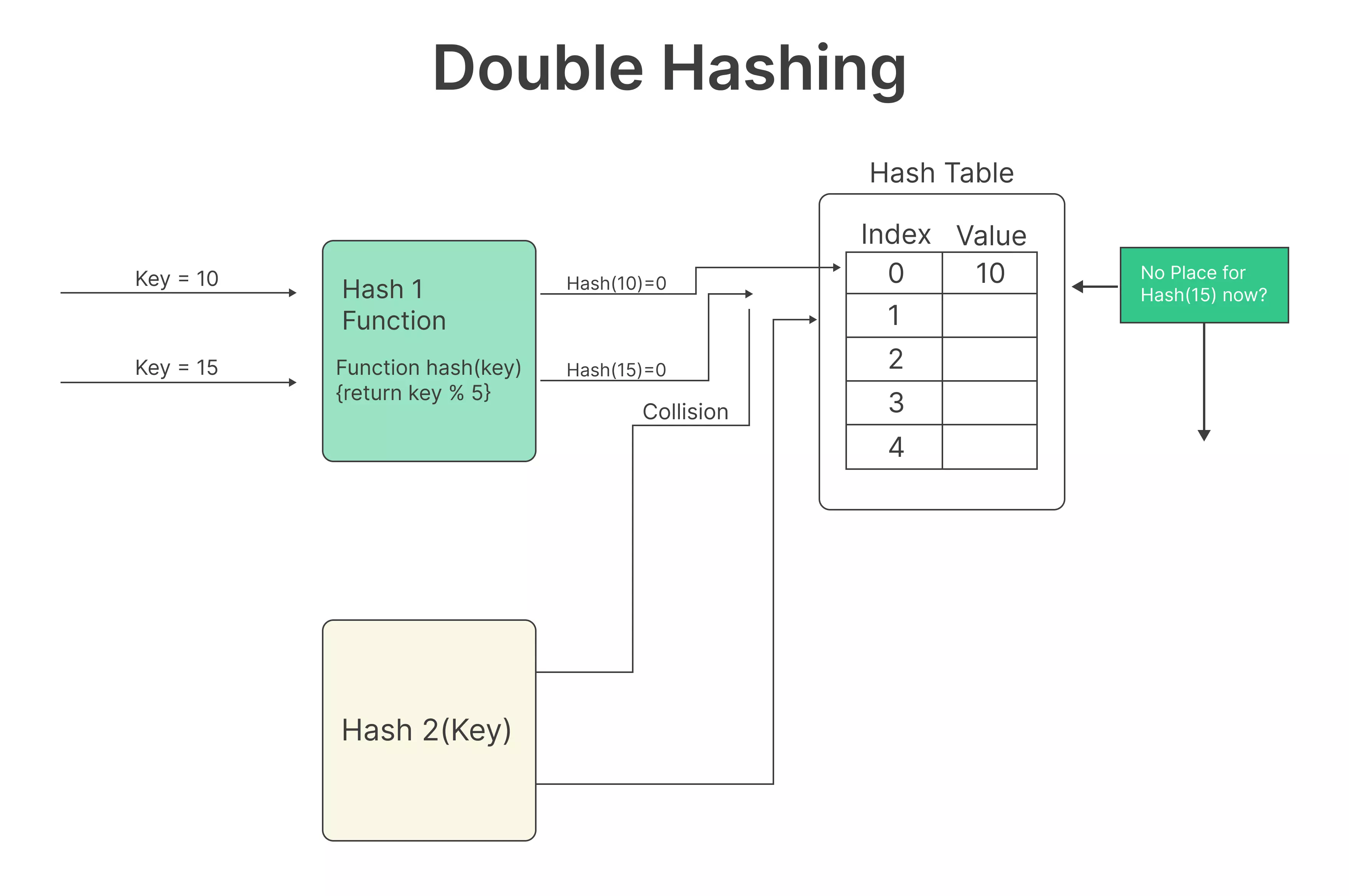# Double Hashing in Python## Introduction to Double Hashing in Python

In the world of data structures and algorithms, one powerful technique that often remains overlooked is double hashing. Double hashing is a collision resolution method that proves to be highly efficient when dealing with hash tables.

Let’s jump into the article to know more about  Double Hashing in Python and how it can be leveraged to optimize your data storage and retrieval processes.

## Understanding Double Hashing in Python

Double hashing is a probing technique used to handle collisions in hash tables. It involves the use of two different hash functions to calculate the next possible location for a key when a collision is encountered. The primary hash function is responsible for calculating the initial hash location, while the secondary hash function guides the search for an available slot in the event of a collision.### Calculating the Initial Hash:

The primary hash function calculates the initial hash index for a given key. This index is the first position where the key should ideally be stored.

### Checking for Collision:

If the calculated index is already occupied by another key, a collision occurs. This is a common occurrence in hash tables due to the limited number of available slots.

### Applying Double Hashing:

When a collision happens, the secondary hash function comes into play. It calculates a new index, which is then added to the initial index, creating a new location for the key.

### Repeating if Necessary:

If the new index is still occupied, the process repeats until an empty slot is found. The secondary hash function ensures that the search pattern doesn’t become repetitive, increasing the chances of finding an empty slot in a reasonable amount of time.### Python Double Hashing Implementation

```# Python code for demonstrating double hashing

class DoubleHashing:
def __init__(self, size):
self.size = size
self.hash_table = [None] * self.size
self.PRIME = 7

def _hash1(self, key):
return key % self.size

def _hash2(self, key):
return self.PRIME - (key % self.PRIME)

def insert(self, key, value):
index = self._hash1(key)

if self.hash_table[index] is None:
self.hash_table[index] = (key, value)
else:
# Collision resolution with double hashing
index2 = self._hash2(key)
i = 1
while True:
new_index = (index + i * index2) % self.size
if self.hash_table[new_index] is None:
self.hash_table[new_index] = (key, value)
break
i += 1

def search(self, key):
index = self._hash1(key)
if self.hash_table[index] is not None and self.hash_table[index] == key:
return self.hash_table[index]
else:
# Search for the key using double hashing
index2 = self._hash2(key)
i = 1
while True:
new_index = (index + i * index2) % self.size
if self.hash_table[new_index] is not None and self.hash_table[new_index] == key:
return self.hash_table[new_index]
i += 1
return None

# Create a hash table
hash_table = DoubleHashing(10)

# Insert data
hash_table.insert(5, "Apple")
hash_table.insert(15, "Banana")
hash_table.insert(25, "Cherry")

# Retrieve data
print(hash_table.search(5))  # Output: "Apple"
```

### Conclusion

In Conclusion, Double Hashing in Python is a powerful technique in data structure design that offers efficient collision resolution and uniform key distribution. By using two independent hash functions, it minimizes clustering and ensures a predictable and reliable performance. When implemented correctly, double hashing can greatly improve the efficiency of hash tables in a wide range of applications.

### Related Banners

Get PrepInsta Prime & get Access to all 200+ courses offered by PrepInsta in One SubscriptionQuestion 1.

How does double hashing differ from linear probing?

Double hashing uses two hash functions to calculate the initial location and step size, while linear probing simply checks the next slot in the hash table.Question 2.

Can you provide an example of double hashing in Python?

The provided Python code demonstrates the implementation of double hashing for handling collisions in a hash table.Question 3.

What are some common use cases for double hashing?

Double hashing is commonly used in scenarios where efficient data retrieval is essential, such as caching mechanisms, database indexing, and symbol tables in compilers.

## Get over 200+ course One Subscription

Courses like AI/ML, Cloud Computing, Ethical Hacking, C, C++, Java, Python, DSA (All Languages), Competitive Coding (All Languages), TCS, Infosys, Wipro, Amazon, DBMS, SQL and others